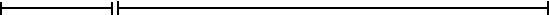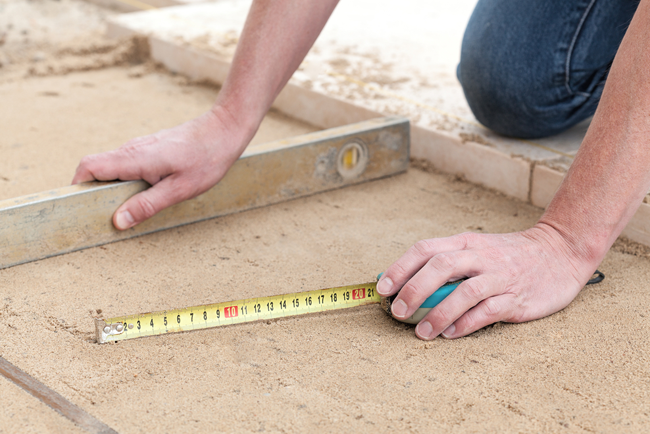## Laying Pavers Part 1 – MeasuringApril 13, 2017Paving an outdoor entertaining area, patio or driveway is simple if you know how. This series of articles will attempt to take you through the process step by step. Each step is easy, there are just a few of them.

Preparation is the key to paving an area that will stand the test of time. If you take the time to prepare your area properly, then your end result will be of a high standard.

### Working Out The Area (square metres) of Your Space

This is the first step in the process. Even though it sounds simple, and it is, if you get it wrong it will impact the rest of process. For example based on your calculations, you will then need to work out excavation quantities, how many bricks/pavers to order, work out quantities of sand and cement. Getting your calculations correct is crucial.

### Brush Up on Your High School Geometry

Most spaces are made up of a variety of shapes, triangle, rectangle, circle and square. Break your space down into a variety of shapes and the work out the area (square meterage) of each individual shape, then add them together.

### Formulas for the Areas of Plain Shapes

Square – S squared, where S is the length of one side.

Triangle – 1/2 B x H half the length of the base multiplied by the vertical height.

Circle – Pi x R squared, where pi is 3.14 multiplied by the radius squared.

Rectangle – L x B Length multiplied by breadth or long side multiplied by the short side.

Ellipse – Pi x A x B where Pi is 3.14, A is the longest possible dimension and B is the shortest possible dimension.

Trapeziums – do have there own formula, however to make it simpler, I usually divide this shape into a rectangle and two triangles.

### Now You Know the Size of Your Space

Once you have calculated the total size of the area you intend to pave, you can begin to estimate the total cost of the project. You now have enough information to work out how much your chosen pavers will cost you, the volume of soil you will need to excavate and potentially the size of the skip bin you will need to hire.